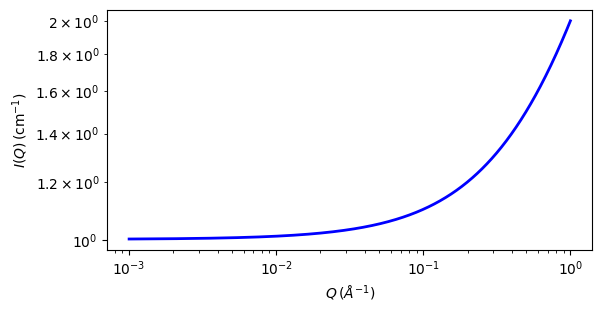# line

Line model

Parameter

Description

Units

Default value

scale

Scale factor or Volume fraction

None

1

background

Source background

cm-1

0.001

intercept

intercept in linear model

cm-1

1

slope

slope in linear model

Å·cm-1

1

The returned value is scaled to units of cm-1 sr-1, absolute scale.

This model calculates intensity using simple linear function

Definition

The scattering intensity $$I(q)$$ is calculated as

$I(q) = \text{scale} (A + B \cdot q) + \text{background}$

Note

For 2D plots intensity has different definition than other shape independent models

$I(q) = \text{scale} (I(qx) \cdot I(qy)) + \text{background}$Fig. 108 1D plot corresponding to the default parameters of the model.

Source

line.py

References

None.

Authorship and Verification

• Author: